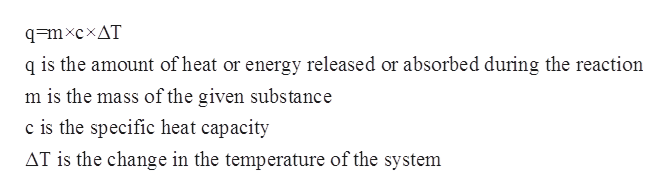# How much heat is required to convert solid ice with a mass of 930 g at a temperature of -10.0 °C to liquid water at a temperature of 59.0 °C? The specific heat of ice is cice = 2100 J/kgK, the specific heat of water is cwater = 4186.8 J/kgK, and the heat of fusion for water is Lf = 334 kJ/kg.

Question

How much heat is required to convert solid ice with a mass of 930 g at a temperature of -10.0 °C to liquid water at a temperature of 59.0 °C? The specific heat of ice is cice = 2100 J/kgK, the specific heat of water is cwater = 4186.8 J/kgK, and the heat of fusion for water is Lf = 334 kJ/kg.

check_circle

Step 1

The specific heat capacity for a given chemical substance or a solution refers to the amount of the heat that is required to increase the temperature of a given substance or system per unit of its mass. The mathematical relationship between specific heat capacity, mass, change in temperature and the heat of the solution is given by the following equation:help_outlineImage Transcriptioncloseq=mxcxAT q is the amount of heat or energy released or absorbed during the reaction m is the mass of the given substance c is the specific heat capacity AT is the change in the temperature of the system fullscreen
Step 2

The initial temperature is given to be -10.0 oC = -283.15 K.

The final temperature is given to be 59 oC =332.15 K.

The specific heat capacity of water is given to 4186.8 J kg-1 K-1.

The specific heat capacity of ice is given to 2100 J kg-1 K-1.

The mass of ice is given to be 930 g=0.93 kg.

This conversion takes place by three steps. In first step, the temperature of the ice raises from -10oC to 0.0oC.

In the first step the heat absorbed during the reaction can be calculated as follows:

Step 3

The heat which is absorbed during the melting...

### Want to see the full answer?

See Solution

#### Want to see this answer and more?

Solutions are written by subject experts who are available 24/7. Questions are typically answered within 1 hour.*

See Solution
*Response times may vary by subject and question.
Tagged in

### Physical Chemistry